Technical Article

# Understanding the Differences Between the N-channel and P-channel Field-effect Transistors (FETs)

July 13, 2021 by Lorenzo Mari

## Learn about the principles of and the operation of the field-effect transistor.

Transistors are essential semiconducting devices in today’s electronic circuits. They can perform two primary functions. Firstly, as their vacuum tube precursor, the triode, they can amplify an electrical signal. Secondly, they can act as switching devices in computers for information processing and storage. Field-effect transistors are semiconductor devices that control the current through an electric field.

Transistors can amplify an electrical signal and act as switching devices. Computers employ the transistor’s switching ability for arithmetic and logical operations and information storage. They use the binary code – numbers written to the base 2 – to express numbers and functions. Series of two states – 0 and 1 – represent numbers.

Transistors within a digital circuit also operate with two states: “on” and “off” – or conducting and nonconducting. “On” corresponds to one binary number state and “off” to the other. Therefore, a collection of circuit elements containing properly switched transistors may characterize a number.

The dipole layers – created by the diffusion process in the FET’s p-n junctions – establish electric fields. These electric fields control the output’s circuit conduction path. This mechanism is the basis of the term field-effect.

Interest in these devices emerges mainly because the input circuit has the characteristics of a single reverse-biased diode. A FET needs a minimal dc input current and has a very high input impedance.

## Types of Field-Effect Transistors

There are three major types of field-effect transistors: the junction field-effect transistor (abbreviated JFET, or simply FET), the metal-oxide-semiconductor field-effect transistor (MOSFET), and the metal-semiconductor field-effect transistor (MESFET).

Other acronyms for metal-oxide semiconductors are MOST (MOS transistor) and IGFET (insulated-gate field-effect transistor).

The MESFET is a modern development that uses high-speed characteristics of gallium arsenide (GaAs) as the base semiconductor material.

Digital applications employ MOSFETs in integrated circuits while JFETs are more common in analog applications.

This article introduces the junction field-effect transistor (JFET) and examines the principles on which these devices operate.

## The Junction Field-effect Transistor

The junction field-effect transistor is a three-terminal device where the voltage applied to one terminal controls the current between the other two – the output circuit current.

There are two types of JFET: n-channel and p-channel. Due to the fact that electrons move faster than holes, n-channel JFETs are more common than p-channel JFETs.

The conduction level in a bipolar junction transistor (BJT) depends on two charge carriers – electrons and holes. The JFET, however, is a unipolar device because its conduction depends uniquely on one type of carrier – electrons (n-channel) or holes (p-channel).

Other essential characteristics of the JFET are:

• Ease of manufacture.
• Small size, adequate for LSI and VLSI digital arrays.
• High input impedance – typically many megohms.
• Less noisy than a BJT.
• No offset voltage at zero drain current.

## The n-Channel Junction Field-effect Transistor

Figure 1 shows a diagram of the n-channel JFET. The geometry shown in this figure simplifies the analysis of the JFET principles.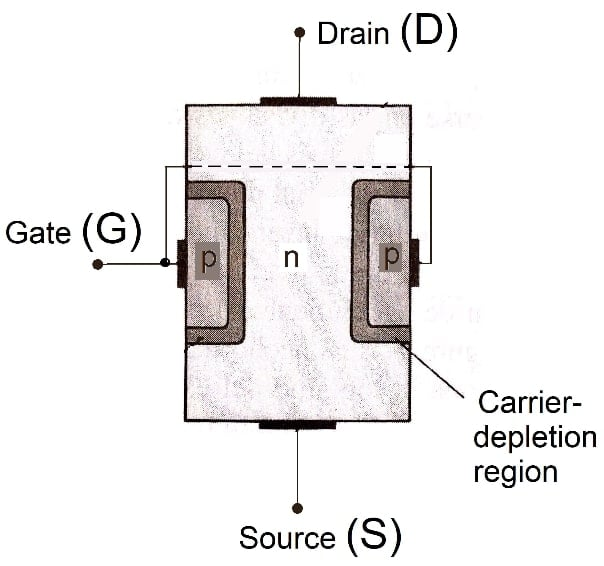##### Figure 1. n-channel JFET.

Both sides of the device have heavily doped p-type regions of acceptor impurities, shaping the gate G. Notice the connection between the two p-type areas and the gate terminal.

The area between the two gate regions is the channel, a structure of n-type material. This narrow semiconductor channel provides a conducting path between the source and the drain. The majority of carriers enter the device through the source S and leave it through the drain D. The source side may be either channel’s end.

The basis of the operation of this structure is to change the resistance between the S and D terminals by adjusting the voltage on gate G.

Figure 2 shows the circuit symbol, and conventions for current direction and voltage polarity, for an n-channel JFET.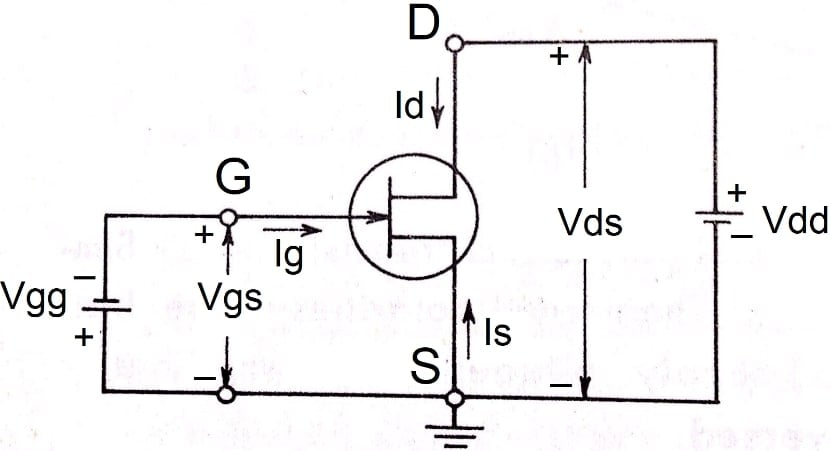##### Figure 2. Circuit symbol and conventions for an n-channel JFET.

The arrowhead at the gate indicates the direction across the junction from the p-type to the n-type JFET.

Is = conventional current entering at S.

Id = conventional current entering at D.

Ig = conventional current entering at G. This is the gate current flow direction, with the gate junction forward-biased.

Vds = drain-to-source applied voltage – positive if d is more positive than s.

Vdd = drain supply voltage (external voltage source).

Vgg = gate supply voltage (external voltage source).

Vgs = gate-to-source applied voltage – positive if g is more positive than s. Vgs is used with a polarity to reverse-bias the p-n junction (Vgs = - Vgg).

For an n-channel JFET, Id and Vds are positive, and Is and Vgs are negative.

## N-channel JFET Operation

Before applying any external voltage to the JFET terminals, there are two p-n junctions under no-bias conditions, creating a carrier-depletion or space-charge region at each junction. The excess carriers (electrons) have been removed or “depleted” from the carrier-depletion regions. Accordingly, the carrier-depletion regions have few free carriers, incapable of supporting conduction (Figure 1).

Figure 3 shows a condition where Vgs = 0 V – with the gate and the source short-circuited – and Vds has a low positive value.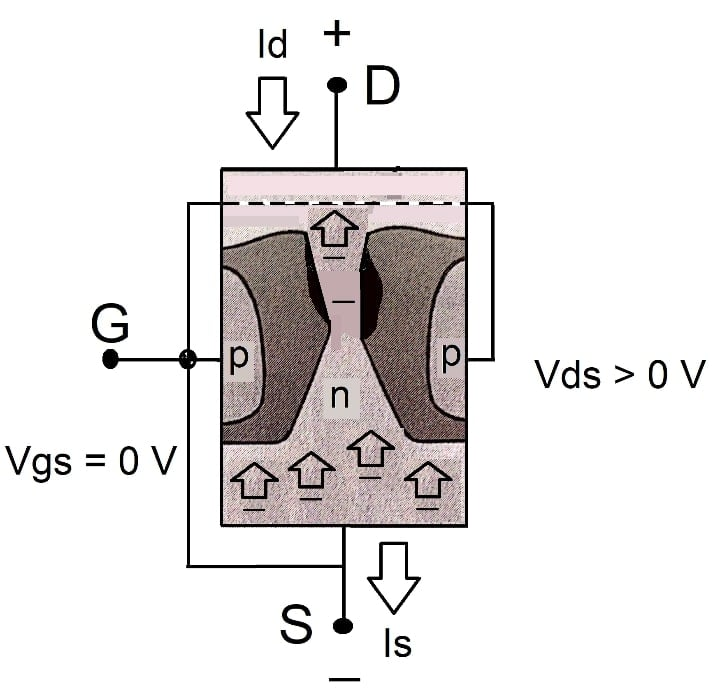##### Figure 3. Vgs = 0 V and Vds > 0 V.

The positive drain terminal attracts the channel´s electrons, generating a current Id.

The current flows in a direction opposite the electron flow by convention; therefore, the current Id enters D.

The current Is has the same magnitude and direction as Id. The positive direction of Is has been defined as entering S.  Therefore, Is is negative under this condition. The current Ig is negligibly small, which is a significant JFET attribute.

The channel’s impedance limits the magnitude of the charge flow in Figure 3.

Note that the carrier-depletion regions get wider while approaching the D side. Assuming a uniformly distributed resistance, the voltage drop in the channel will increase from 0 V at S to Vds at D. Then, the p-n junctions will be increasingly reverse-biased from S to D. As the reverse bias across the junctions increases, so does the thickness of the region of immobile uncovered charges.

Uncovered charges are the bound charges in the carrier-depletion region – negative ions on the p-type side and positive ions on the n-type side. They create a dipole layer of charge at the junction. The electric field lines originating on the positive ions and terminating on the negative ions are the source of the voltage drops across the junctions. The term field-effect describes this mechanism because current control results from the extension, with growing reverse bias, of the field associated with the region of uncovered charges.

Figure 4 shows a source-drain characteristic of an n-channel JFET, giving Id vs. Vds, with Vgs = 0.##### Figure 4. Id vs. Vds, Vgs = 0 V.

When Id = 0, the channel is open. Increasing the value of Vds to a few volts, the current rises linearly following ohm’s law; this is why the plot grows nearly as a straight line – the resistance is constant, and the n-type JFET acts as a simple semiconductor resistor.

Vds increments broaden the depletion regions, and the conducting portion of the channel begins to shrink – progressively decreasing the channel’s effective width and increasing its resistance.

Due to the ohmic drop along the channel, the shrinking is not uniform but is more evident at distances farther from the source.

Increasing Vds to a level where it appears that the two depletion regions would touch, as shown in Figure 5, results in a condition referred to as the pinch-off. In this condition, Vds = Vp – the pinch-off voltage.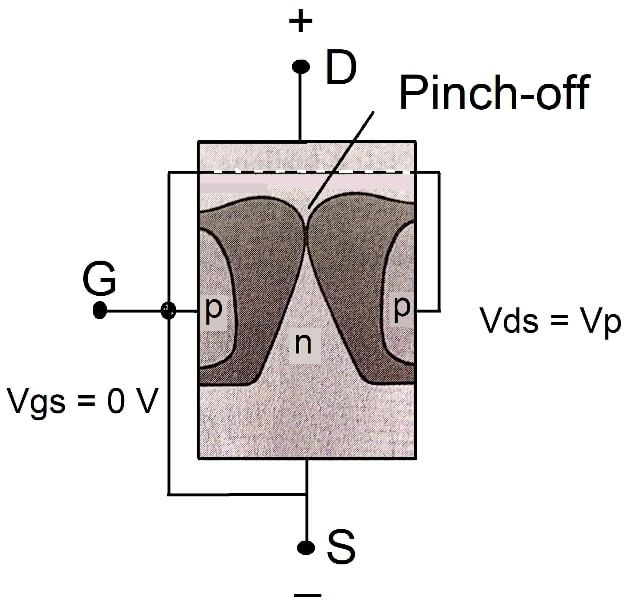##### Figure 5. Vgs = 0 V, Vds = Vp.

After Vds reaches Vp, the curve in Figure 4 begins to level off. The current Id approaches a constant value – the saturation level – shown as Idss (saturated short-circuit drain current). The channel’s resistance will tend to infinite ohms in the curve’s horizontal region. At pinch-off, there is a tiny channel with a high-density current.

In Figure 5, the channel is just at the threshold of pinch-off at the drain end. Increasing Vds beyond Vp lengthens the contact of the two depletion regions along the channel, but Idss remains constant. Under this condition, the JFET acts as a current source.

The channel cannot close completely at pinch-off value, reducing Id to zero – instead, Id retains the saturation level shown in Figure 4.  If that were the case, the ohmic drop providing the reverse bias along the p-n junction would be absent – losing the depletion region that caused pinch-off.

## Vgs Providing Additional Reverse Bias

The gate to source voltage (Vgs) controls the JFET. Setting the gate terminal at gradually lower potential levels than the source produces a family of Id vs. Vds curves, with Vgs as a parameter. Figure 6 shows typical curves for an n-channel JFET.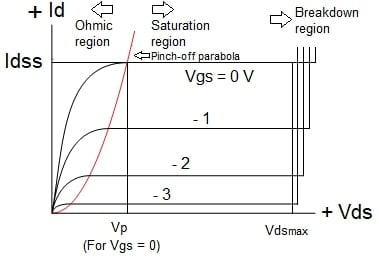##### Figure 6. Characteristics of an n-channel JFET.

Applying a gate voltage Vgs in the direction providing additional reverse bias establishes depletion regions similar to Vgs = 0, but at lower levels of Vds, causing pinch-off – and saturation level – to occur at smaller values of Vds. Then, Vgs aids Vds in producing pinch-off. With a reduced value of Vds across the channel, pinch-off occurs at a smaller drain current.

Applying a little positive voltage to the gate – which is in the direction of forwarding bias – the value of Vds required to produce pinch-off increases, with a corresponding rise in the drain current at which pinch-off appears. It is not convenient to apply high positive voltages to avoid undesirable currents at the gate terminal.

As before, each characteristic curve has an ohmic region for small values of Vds – Id proportional to Vds – and a constant current region for large values of Vds – where Id marginally responds to Vds. When Vds rises beyond the pinch-off level, the pinched-off channel limits the current to the magnitude existing when the pinch-off occurred.

Figure 6 also shows that the pinch-off voltage drops parabolically, and the magnitude of the saturation current – for large values of Vds – decreases as Vgs gets more negative.

Vgs = - Vp produces a saturation level with a minimal Id current, and we may conclude that the transistor is “off.”

Note that when Vds reaches higher magnitudes, the curves suddenly rise to levels that seem infinite. This rise denotes the avalanche breakdown across the p-n junctions; only the output circuit elements limit the current through the channel under this condition.

The maximum voltage to be applied between any two JFET terminals is the lowest voltage that will cause avalanche breakdown across the p-n junction. Figure 6 shows that avalanche occurs at lower Vds values, as the gate is more reverse biased. It happens because the reverse-bias gate voltage (Vgs) adds to the drain voltage (Vds), escalating the actual voltage across the p-n junction.

## The P-Channel Junction Field-effect Transistor

The p-channel JFET is the reversal of the n-channel, with the p- and n-type materials structured as shown in Figure 7.##### Figure 7. p-channel JFET.

Figure 8 shows the circuit symbol and polarity conventions for a p-channel JFET.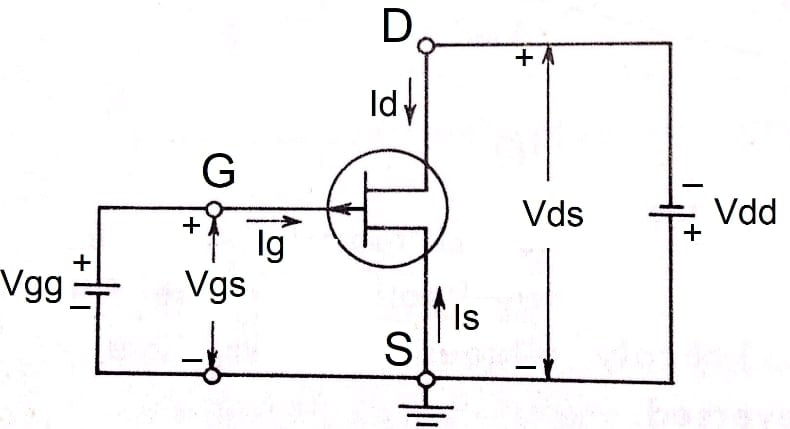##### Figure 8. Circuit symbol and conventions for a p-channel JFET.

The p-channel JFET has opposite current directions and voltage polarities when compared to the n-channel JFET; Id and Vds are negative and Is and Vgs are positive. Therefore, increasing positive voltages from gate to source will constrict the channel.

Figure 8 keeps the references used in Figure 2 for the n-channel JFET, for the directions of the current's Id, Is, and Ig, and for the polarities of the voltages Vds and Vgs. The gate junction’s arrow points in the opposite direction (showing a path from the p-type to the n-type).

Figure 9 shows typical curves for a p-channel JFET.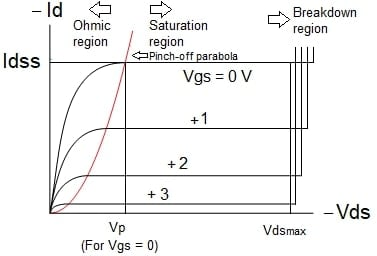##### Figure 9. Characteristics of a typical p-channel JFET.

Figure 9 shows positive values for Vgs and negative values for Vds – the source is at a higher potential than the drain.

Again, the curves abruptly rise to extreme levels at high negative values of Vds, suggesting avalanche breakdown.

Note:

The two types of transistors – n-channel and p-channel – are said to be complementary. An n-channel JFET in a circuit may be replaced by a p-channel transistor of similar rating, reversing the power-supply (Vdd) polarity and all polarity-sensitive devices, such as electrolytic capacitors and diodes.

The three electrical terminals of the JFET are the drain (D), the source (S), and the gate (G).

The majority of carriers flow from the source through the channel to the drain. The channel may be either an n- or p-type crystal.

The gate-source voltage controls the electric field – and the drain current (Id) in the channel. The gate junctions typically have a reverse-bias voltage across them, resulting in negligible currents flowing at the gate terminal.

Considering an n-channel device, if the gate-source voltage Vgs = 0 V and the drain-to-source voltage Vds is positive, electrons drift through the channel due to the electric field. The drain current Id is proportional to the channel’s resistance, providing that Vds is small.

The width of the carrier-depletion regions at the p-n junctions depends on the voltage Vgs. Variations in Vgs modify the channel dimensions. The carrier-depletion regions act as a valve to control the amount of current in the channel and, subsequently, the magnitude of the current Id.

The positive drain voltage reverse biases the p-n junctions, mainly near the channel’s drain end. When Vds reaches the pinch-off voltage (Vp), the channel thickness reduces to nearly zero at a point close to the drain end. Id is not zero when Vds = Vp because this voltage still exists between the pinch-off point and the source end, and the resulting electric field accelerates the carriers through the channel to the drain end.

For values Vds > Vp, the depletion region thickness increases between the gate and the drain, with little change between the pinch-off point and the source. As a result, the additional voltage appears across the depletion region with little change in the electric field along the channel. The consequence is a constant Id value.

The current measured under Vds = Vp and Vgs = 0 V is the saturated short circuit drain current (Idss).

The JFET typically operates with Vds > Vp and reverse bias applied to the gate. When Vds + Vgs > Vp, Id is almost independent of Vds.

Avalanche breakdown arises at the p-n junction for high values of Vds.

The input impedance of a JFET is very high but drops sharply when avalanche breakdown occurs.

The p-channel JFET has a p-type channel and n-type gates. It operates like the n-channel transistor but with reversed voltage and current polarities.